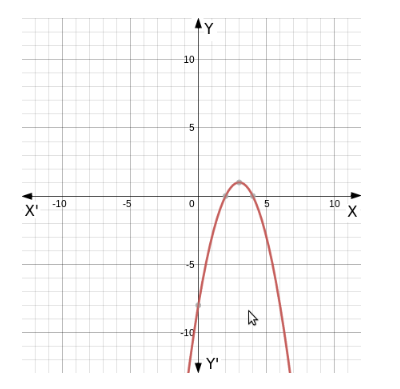# The function f : A→B defined by

Question:

The function $f: A \rightarrow B$ defined by $f(x)=-x^{2}+6 x-8$ is a bijection if

(a) $A=(-\infty, 3]$ and $B=(-\infty, 1]$

(b) $A=[-3, \infty)$ and $B=(-\infty, 1]$

(c) $A=(-\infty, 3]$ and $B=[1, \infty)$

(d) $A=[3, \infty)$ and $B=[1, \infty)$

Solution:

(a) $A=(-\infty, 3]$ and $B=(-\infty, 1]$

$f(x)=-x^{2}+6 x-8$, is a polynomial function

And the domain of polynomial function is real number.\

$\therefore x \in R$

$f(x)=-x^{2}+6 x-8$

$=-\left(x^{2}-6 x+8\right)$

$=-\left(x^{2}-6 x+9-1\right)$

$=-(x-3)^{2}+1$

Maximum value of $-(x-3)^{2}$ woud be 0

$\therefore$ Maximum value of $-(x-3)^{2}+1$ woud be 1

$\therefore f(x) \in(-\infty, 1]$We can see from the given graph that function is symmetrical about $x=3$

\& the given function is bijective.

So, $x$ would be either $(-\infty, 3]$ or $[3, \infty)$

The correct option which satisfy A and B both is:

$A=(-\infty, 3]$ and $B=(-\infty, 1]$